# Classification of isolated singularities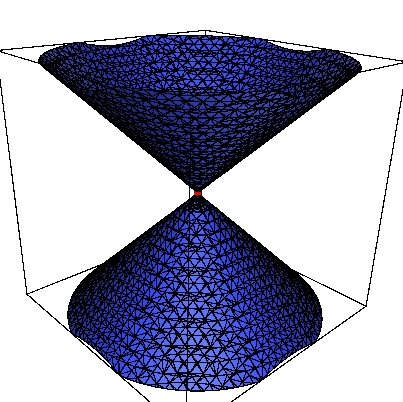## A1 Singularity

First type in the classification of isolated singularities, with real signature --. It is a simple cone. The equation with coefficient signsature ++, also defines a A1 singularity, but it is an isolated point, over the real.
Equation: x^2-y^2-z^2## A2-- singularity

Second type 2 in the singularity classification, with real signature --.
Equation: x^3-y^2-z^2## A2+- singularity

Second type 2 in the singularity classification, with real signature +-.
Equation: x^3+y^2-z^2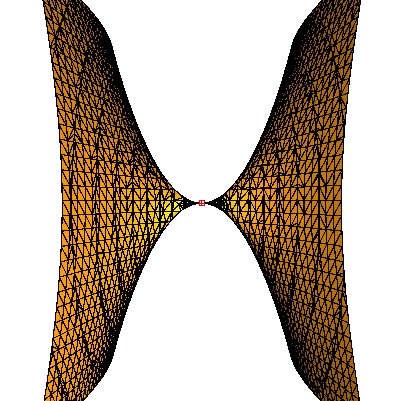## A3--

Equation: x^4-y^2-z^2;## A3+-

Equation: x^4+y^2-z^2;

## A3++

Equation: x^4+y^2+z^2;

## A4 singularity

A4 singularity with coefficient signs - -.
Equation: x^5-y^2-z^2## A4 singularity

A4 singularity with coefficient signs +-.
Equation: x^5+y^2-z^2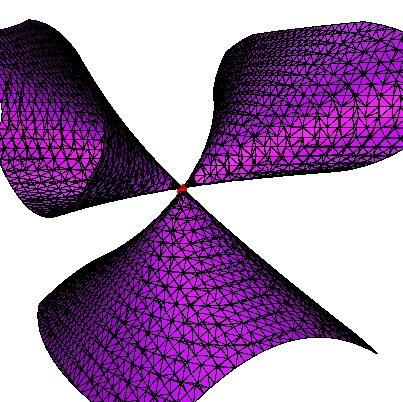## D4 singularity

D4 singularity with coefficient signs --.
Equation: x^2*y-y^3-z^2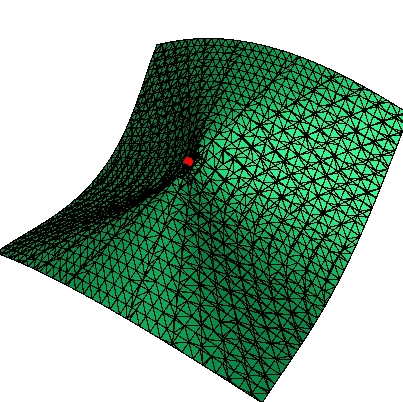## D4 singularity

D4 singularity.
Equation: x^2*y+y^3-z^2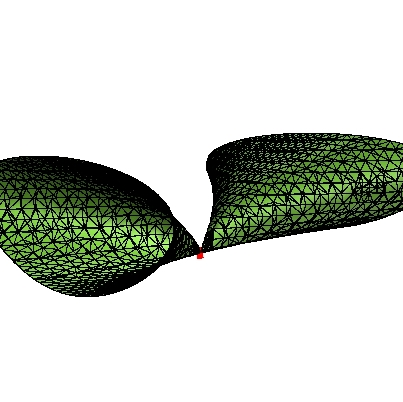## D5 singularity

D5 singularity with coefficient signs --.
Equation: x^2*y-y^4-z^2## D5 singularity

D5 singularity with coefficient signs +-.
Equation: x^2*y+y^4-z^2## D6 singularity

D6 singularity with coefficient signs --.
Equation: x^2*y-y^5-z^2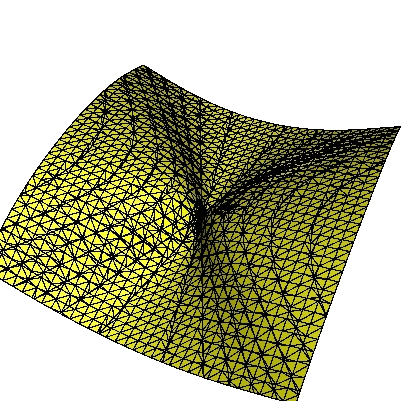## D6 singularity

D6 singularity with coefficient signs +-.
Equation: x^2*y+y^5-z^2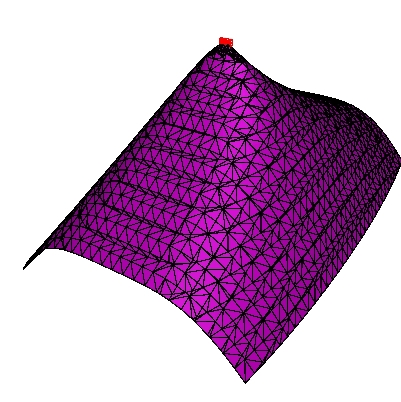## A6 singularity

A6 singularity with coefficient signs --.
Equation: x^3-y^4-z^2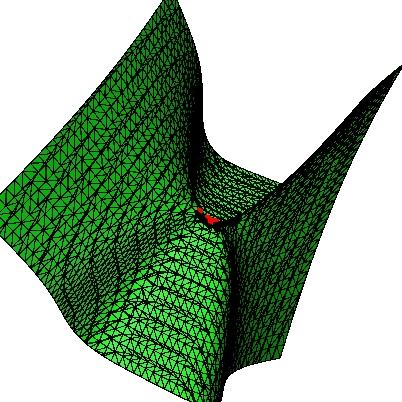## E6 singularity

E6 singularity with coefficient signs +-.
Equation: x^3+y^4-z^2## E7 Singularity

E7 singularity.
Equation: x^3-x*y^3-z^2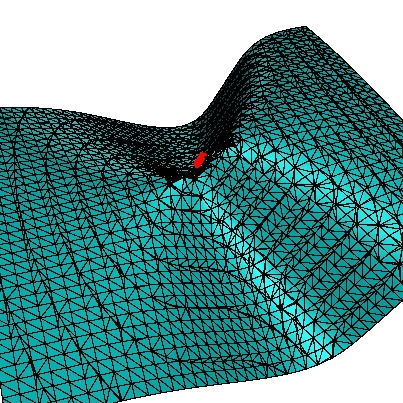## E8 singularity

E8 singularity.
Equation: x^3-y^5-z^2

## monoidnot

Equation: y^2*z-x^3+x*z+z^4

## monoidt3310

Equation: -x*y*z+x^3-x^2*y+y^3-y*z^3+x^2*z^2-x^3*z+x^4

## monoidt334

Equation: y^2*x-x^2*z-x^3-z^4

## monoidt339

Equation: -x*y*z+x^3+y^3-y*z^3+x^2*z^2

## monoidt344

Equation: -x^3+y^2*z-z^4

## monoidt355

Equation: -x^3+y^2*z-y*z^3